# defaults
.c <- .4
.d <- .2
.s <- .4
.v <- .2
.delta <- .4
.A_cooptn <- 1.5
.A_nocoop <- 1
.A_defect <- 1.4
.c_range <- c(.3, .4, .5)
.v_range <- c(0, .2, .4)
.delta_range <- c(.2, .4, .6)

# 1 Set up

## 1.1 Capital evolution

We define a function to describe the evolution of capital and utility given parameters.

# Total welfare if you pay cost c each period, form production, and produce according to A k ^ a
# Evolution of capital and instantaneous utility
# Can be used for path segment for regime specific A and starting k
k_path <- function(k, A = .A_nocoop,  c = 0,  d = .d, a = .5, s = .s, max = 100, delta = .5){

# Initiate
f  <- function(k) max(0, A*k^a - c)
ys <- list(f(k))
ks <- list(k)
us <- list((1-s)*(ys[] - c))

if(max>1)
for(j in 2:max){

ks[[j]] <- max(0, ks[[j-1]]*(1-d) + s*(ys[[j-1]]))
ys[[j]] <- f(ks[[j]])
us[[j]] <- (1-s)*ys[[j]]
}

k <- unlist(ks)
k_end <- c(k[-1],  max(0, tail(k,1)*(1-d) + s*(A*tail(k,1)^a-c)))

data.frame(t = 1:max, k = k, y = unlist(ys), u = unlist(us), k_end = k_end)

}

# k_path(1, max = 1)
# k_path(1, max = 3)

# Welfare function
# Welfare during a regime starting at k with path defined for that regime
W <- function(k, delta = .5, ...){
H <- k_path(k, ...)
sum((delta^(0:(nrow(H)-1))) * H$u) } # Illustration of concatenation of paths # Note: when adding the second chunk we need to input the ending capital k_path(1, max = 4) %>% kable(caption = "Sample evolution") Sample evolution t k y u k_end 1 1.000000 1.000000 0.6000000 1.200000 2 1.200000 1.095445 0.6572671 1.398178 3 1.398178 1.182446 0.7094675 1.591521 4 1.591521 1.261555 0.7569329 1.777839 If parameters change, a complex path can be defined by concatenating two paths, with appropriate discounting. • W(1, max = 4) = 1.200617 • W(1, max = 2) + .25*W(k = k_path(1, max = 2)$k_end, max = 2) = 1.200617

## 1.2 Figuring out the cutoff when cooperation becomes sustainable?

We have a function to search over $$k$$ values to assess when, starting at $$k$$, players prefer general cooperation to produce $$A$$ at cost $$c$$ rather than defection.

# Willing to start cooperating
will_cooperate <- function(k, c = .c, s = .s, d = .d, a = .5,
A_cooptn = .A_cooptn,
A_nocoop = .A_nocoop,
A_defect = .A_defect,
delta    = .delta,
...) {
# Defector's capital
k2_defect <- (1-d)*k + s*A_defect*k^a

((1-s)*A_defect*k^a +       # This period cheater payoff + future anarchy
delta*W(k2_defect, delta = delta, c = 0, s = s, a = a, d = d, A = A_nocoop, ...)) <=

W(k, delta = delta, c = c, s = s, a = a, d=d, A = A_cooptn, ...)
}

coopertion_cutoff <- function(kmax = 8, fine =.01, ...){
# large kmax as we can go beyond the steady state of no cooperation when violence is an option
ks <- seq(0, kmax, fine)
df <- data.frame(k = ks, will = sapply(ks, will_cooperate, ...))
if(all(!df$will)) return(NA) df$k[df$will] } print(paste("sample cutoff:", coopertion_cutoff())) ##  "sample cutoff: 5.68" ## 1.3 Decision Rules The enforcer is willing to start enforcing the collective action when this is better than no cooperation given equilibria in which (a) it might go forever or (b) it might transition to cooperation if others get wealthy enough. • We focus on a given point when everyone has capital $$k$$ • Assess who will accept a transition to a violence equilibrium now given possible later shift to cooperate equilibrium. • Assess who will accept a transition to an all cooperate equilibrium now • Value of commitment to natural transition also assessed # for these calculations max is assumed large: at any point we imagine discounted payoffs far into the future values <- function(k, c = .c, s = .s, d = .d, a = .5, A_cooptn = .A_cooptn, A_nocoop = .A_nocoop, A_defect = .A_defect, delta = .delta, v = .v, max = 400, coop_cut = NULL, ...) { # capital cutoff point when all cooperate is sustainable if(is.null(coop_cut)) coop_cut <- coopertion_cutoff(c = c, s = s, d = d, a = a, delta = delta, A_cooptn = A_cooptn, A_defect = A_defect) # Violence paths, from here cit_path_v <- k_path(k, A = A_defect, c = c, s = s, d = d, a = a, delta = delta, max = max) enf_path_v <- k_path(k, A = A_defect, c = v, s = s, d = d, a = a, delta = delta, max = max) # Anarchy path, from here path_n <- k_path(k, A = A_nocoop, c = 0, s = s, d = d, a = a, delta = delta, max = max) # Cooperation path, from here path_c <- k_path(k, A = A_cooptn, c = c, s = s, d = d, a = a, delta = delta, max = max) # Length of violence period, from now l_violence <- ifelse(any((cit_path_v$k_end >= coop_cut)), min(cit_path_v$t[cit_path_v$k_end >= coop_cut]), max(cit_path_v$t)) # capital at end of violence period k_enf_at_end <- enf_path_v %>% filter(t == l_violence) %>% pull(k_end) k_cit_at_end <- cit_path_v %>% filter(t == l_violence) %>% pull(k_end) # length of natural transition path and capital at end t_AC <- ifelse(any((path_n$k_end >= coop_cut)), min(path_n$t[path_n$k_end >= coop_cut]), max)

k_n  <- path_n %>% filter(t == t_AC)  %>% pull(k_end)

# Payoff to enforcer from temp violence
U_enf_v <- W(k, delta = delta, c = v, A = A_defect, max = l_violence) +
W(k_enf_at_end, delta = delta, c = c, d=d, a=a, s=s, A = A_cooptn,   max = max - l_violence) *
delta^l_violence

# Payoff to citizen from temp violence
U_cit_v <- W(k, delta = delta, c = c, d=d, a=a, s=s, A = A_defect, max = l_violence) +
W(k_cit_at_end, delta = delta, c = c, d=d, a=a, s=s, A = A_cooptn,   max = max - l_violence) *
delta^l_violence

# Payoff to enforcer from permanent violence
U_enf_vv <- W(k, delta = delta, c = v, d=d, a=a, s=s, A = A_defect, max = max)

# Payoff to citizen from permanent violence
U_cit_vv <- W(k, delta = delta, c = c, d=d, a=a, s=s, A = A_defect, max = max)

# Payoff from  natural transition to cooperation
U_cit_n <- W(k,   delta = delta, c = 0, d=d, a=a, s=s, A = A_nocoop, max = t_AC) +
W(k_n, delta = delta, c = c, d=d, a=a, s=s,, A = A_cooptn,   max = max - t_AC) *
delta^t_AC

# Payoff from anarchy
U_anarchy <- W(k,   delta = delta, c = 0, d=d, a=a, s=s, A = A_nocoop, max = max)

# Payoff from all cooperate path
U_cit_c <- W(k, delta = delta, c = c, d = d, a = a, s = s , A = A_cooptn, max = max)

# Payoff to citizen from *defecting* from a cooperation path
# =
# Payoff to enforcer from *defecting*  (not investing in v)
U_enf_df1 <-
W(k, delta = delta, c = 0, d = d, a = a, s = s, A = A_defect, max = 1) +
W((1-d)*k + s * A_defect*k^a,
delta = delta, c = 0, d=d, a=a, s=s, A = A_nocoop,   max = max - 1) * delta

# Payoff to enforcer from *defecting* this time (investing in c!)
U_enf_df2 <-
W(k, delta = delta, c = c, d=d, a=a, s=s, A = A_cooptn, max = 1) +
W((1-d)*k + s * (A_cooptn*k^a - c),
delta = delta, c = 0, d=d, a=a, s=s, A = A_nocoop,   max = max - 1) *
delta

# Returns
c(k= k,
U_enf_v = U_enf_v,
U_cit_v= U_cit_v,
U_enf_vv = U_enf_vv,
U_cit_vv = U_cit_vv,
U_anarchy = U_anarchy,
U_cit_n = U_cit_n,
U_cit_c = U_cit_c,
U_enf_df1 = U_enf_df1,
U_enf_df2 = U_enf_df2,
l_violence = l_violence,
t_AC=t_AC
)
}

Given k, can a violent period be sustained in equilibrium starting now?

• Note that provider wants the transition before the point that others are willing to accept
• But provider still needs to be incentivized not to shirk – i.e. be willing to pay $$v$$ when all others cooperate.
• Note that a provider doing destruction out of equilibrium is not optimal since he does no better than all defect and does worse because he pays for violence
values_given_k <- sapply(c(1, 1.5, 2, 2.5, 3, 3.5, 4), values) %>% t() %>% data.frame

values_given_k %>%  kable(digits = 2)
k U_enf_v U_cit_v U_enf_vv U_cit_vv U_anarchy U_cit_n U_cit_c U_enf_df1 U_enf_df2 l_violence t_AC
1.0 1.20 0.85 1.20 0.85 1.06 1.06 0.97 1.33 0.89 33 400
1.5 1.50 1.16 1.50 1.16 1.27 1.27 1.30 1.59 1.17 31 400
2.0 1.75 1.41 1.75 1.41 1.45 1.45 1.58 1.82 1.41 28 400
2.5 1.98 1.64 1.98 1.64 1.61 1.61 1.82 2.02 1.62 26 400
3.0 2.18 1.84 2.18 1.84 1.75 1.75 2.03 2.19 1.81 24 400
3.5 2.36 2.02 2.36 2.02 1.88 1.88 2.23 2.36 1.98 22 400
4.0 2.53 2.20 2.53 2.20 2.00 2.00 2.42 2.51 2.14 19 400

Function to find cutoffs.

# Where is the cutoff for the start of enforced cooperation?

find_cutoff_start <- function(kmax = 10,
fine = .2,
c = .c,
s = .s,
d = .d,
a = .5,
A_cooptn = .A_cooptn,
A_nocoop = .A_nocoop,
A_defect = .A_defect,
delta    = .delta,
v = .v,
coop_cut = NULL,
max = 200,
improvement_for_c = FALSE,
improvement_for_e = FALSE
) {
coop_cut   <- coopertion_cutoff(
c = c, s = s, d = d, a = a, delta = delta, A_cooptn = A_cooptn, A_defect = A_defect, A_nocoop=A_nocoop
)

# Hack for no equilibrium case
if(is.na(coop_cut)) coop_cut <- max + 1

values_given_k <- sapply(seq(0.01, kmax, fine), values, coop_cut = coop_cut,
c = c, s = s, d = d, a = a, v = v, delta = delta, A_cooptn = A_cooptn, A_defect = A_defect
) %>% t() %>% data.frame

# Allow equilibrium selection to depend on citizen valuation
.cut_transition_to_temp_violence = values_given_k %>%
filter((U_enf_v > U_enf_df1) & (U_enf_v > U_enf_df2))
if(improvement_for_e) .cut_transition_to_temp_violence <-
filter(.cut_transition_to_temp_violence, U_enf_v > U_anarchy)
if(improvement_for_c) .cut_transition_to_temp_violence <-
filter(.cut_transition_to_temp_violence, U_cit_v > U_anarchy)

# Allow equilibrium selection to depend on citizen valuation
.cut_transition_to_perm_violence = values_given_k %>%
filter((U_enf_vv > U_enf_df1) & (U_enf_vv > U_enf_df2))
if(improvement_for_e) .cut_transition_to_perm_violence <-
filter(.cut_transition_to_perm_violence, U_enf_vv > U_anarchy)
if(improvement_for_c) .cut_transition_to_perm_violence <-
filter(.cut_transition_to_perm_violence, U_cit_vv > U_anarchy)

list(
transitions =c(
# Point at which violence preferred (assuming citizen won't defect) with no shift to all cooperate
# # enforcer prefers enforcement payoff to anarchy
# # citizens prefers enforcement payoff to anarchy
# enforcer prefers enforcement payoff to defecting down
cut_transition_to_temp_violence =
.cut_transition_to_temp_violence %>% pull(k) %>% min,

# Point at which not tempted to defect
cut_transition_to_all_coop = coop_cut,
cut_transition_to_all_coop_check = values_given_k %>%
filter(U_cit_c >= U_enf_df1) %>% pull(k) %>% min,

# Point at which violence preferred (assuming citizen won't defect) with no shift to all cooperate
cut_transition_to_perm_violence =
.cut_transition_to_perm_violence %>% pull(k) %>% min
),
values_given_k = values_given_k
)

}

Sample cutoffs:

find_cutoff_start(delta = .8, fine = .1, c = .5, v = .2)$transitions %>% kable(caption = "delta = .8, fine = .1, c = .5, v = .2") delta = .8, fine = .1, c = .5, v = .2 x cut_transition_to_temp_violence 0.11 cut_transition_to_all_coop 0.98 cut_transition_to_all_coop_check 1.01 cut_transition_to_perm_violence 0.11 # Should prefer coop to perm violence when costs the same find_cutoff_start(delta = .6, fine = .1, c = .5, v = .5)$transitions %>%
kable(caption = "delta = .6, fine = .1, c = .5, v = .5")
delta = .6, fine = .1, c = .5, v = .5
x
cut_transition_to_temp_violence 7.11
cut_transition_to_all_coop 3.53
cut_transition_to_all_coop_check 3.61
cut_transition_to_perm_violence 8.71
find_cutoff_start(delta = .01, fine = .1, c = .5, v = .2)$transitions %>% kable(caption = "delta = .01, fine = .1, c = .5, v = .2") delta = .01, fine = .1, c = .5, v = .2 x cut_transition_to_temp_violence Inf cut_transition_to_all_coop 201 cut_transition_to_all_coop_check Inf cut_transition_to_perm_violence Inf find_cutoff_start( delta = .4, fine = .1, c = .4, v = 0)$transitions %>%
kable(caption = "delta = .4, fine = .1, c = .4, v = 0")
delta = .4, fine = .1, c = .4, v = 0
x
cut_transition_to_temp_violence 0.11
cut_transition_to_all_coop 5.68
cut_transition_to_all_coop_check 5.71
cut_transition_to_perm_violence 0.11
find_cutoff_start( delta = .4, fine = .1, c = .4, v = .1)$transitions %>% kable(caption = "delta = .4, fine = .1, c = .4, v = .1") delta = .4, fine = .1, c = .4, v = .1 x cut_transition_to_temp_violence 0.51 cut_transition_to_all_coop 5.68 cut_transition_to_all_coop_check 5.71 cut_transition_to_perm_violence 0.51 find_cutoff_start(delta = .4, fine = .1, c = .c, v = .1)$values_given_k %>%
head %>% kable(digits = 2)
k U_enf_v U_cit_v U_enf_vv U_cit_vv U_anarchy U_cit_n U_cit_c U_enf_df1 U_enf_df2 l_violence t_AC
0.01 0.06 -0.24 0.06 -0.24 0.18 0.18 -0.24 0.22 NaN 400 400
0.11 0.45 -0.17 0.45 -0.17 0.42 0.42 -0.11 0.52 0.00 46 400
0.21 0.63 0.06 0.63 0.06 0.54 0.54 0.13 0.68 0.18 40 400
0.31 0.77 0.21 0.77 0.21 0.64 0.64 0.29 0.80 0.31 38 400
0.41 0.89 0.34 0.89 0.34 0.72 0.72 0.43 0.90 0.42 37 400
0.51 0.99 0.45 0.99 0.45 0.79 0.79 0.54 0.99 0.52 36 400

Function to find equilibrium paths given utility profiles. We seek specifically paths for four equilibria, in which:

• players defect until all willing to cooperate (natural transition)
• players defect until all willing to accept violence
• players defect until all willing to accept violence and continue until all willing to cooperate (not all prefer to cooperate)
• players always defect

These are based on utilities from each path given $$k$$.

k_start = .1; kmax = 10; fine = .2; c = .5; s = .s; d = .d; a = .5; A_cooptn = .A_cooptn; A_nocoop = .A_nocoop; A_defect = .A_defect; delta    = .6; v = .v; coop_cut = NULL;  max = 200

paths <- function(k_start = .05,
kmax = 10,
fine = .1,
c = .c,
s = .s,
d = .d,
a = .5,
A_cooptn = .A_cooptn,
A_nocoop = .A_nocoop,
A_defect = .A_defect,
delta    = .delta,
v = .v,
coop_cut = NULL,
max = 200,
improvement_for_c = FALSE,
improvement_for_e = FALSE) {

cutoffs <- find_cutoff_start(
kmax = kmax, fine = fine,
c = c, s = s, d = d, a = a,
A_cooptn = A_cooptn, A_nocoop = A_nocoop, A_defect = A_defect,
delta = delta, v = v,
coop_cut = coop_cut,
improvement_for_c = improvement_for_c,
improvement_for_e = improvement_for_c,
max = 200)

# Find transitions; replace infinite with finite endpoint
transitions <- cutoffs$transitions transitions[transitions==Inf] <- max + 1 # Check in out timing for k: k in or k end? path_anarchy <- k_path(k_start, A = A_nocoop, c = 0, s = s, d = d, a = a, delta = delta, max = max)%>% mutate(eq = "A", i = "citizens") # Transition only when all ready to path_AC <- filter(path_anarchy, (k <= transitions["cut_transition_to_all_coop"])) path_AC <- path_AC %>% rbind(k_path(max(path_AC$k_end), A = A_cooptn, c = c, s = s,
d = d, a = a, delta = delta, max = max - nrow(path_AC)) %>%
mutate(eq = "C",  i = "citizens")) %>%
mutate(t = 1:n())

# Transition to, and stay in, violent equilibria
path_perm_violence_c <- filter(path_anarchy, (k <= transitions["cut_transition_to_perm_violence"]))
path_perm_violence_c <- path_perm_violence_c %>%
rbind(k_path(max(path_perm_violence_c$k_end), A = A_defect, c = c, s = s, d = d, a = a, delta = delta, max = max - nrow(path_perm_violence_c)) %>% mutate(eq = "V", i = "citizens")) %>% mutate(t = 1:n()) path_perm_violence_e <- filter(path_anarchy, (k <= transitions["cut_transition_to_perm_violence"])) path_perm_violence_e <- path_perm_violence_e %>% rbind(k_path(max(path_perm_violence_e$k_end), A = A_defect, c = v, s = s,
d = d, a = a, delta = delta, max = max - nrow(path_perm_violence_e)) %>%
mutate(eq = "V",  i = "agent")) %>%
mutate(t = 1:n(),  i = "agent")

# Transition to, and out of, violent equilibria
path_temp_violence_c <- path_anarchy %>%
select(-i) %>% filter((k <= transitions["cut_transition_to_temp_violence"]))
path_temp_violence_c <- path_temp_violence_c %>%
rbind(k_path(max(path_temp_violence_c$k_end), A = A_defect, c = c, s = s, d = d, a = a, delta = delta, max = max - nrow(path_temp_violence_c)) %>% mutate(eq = "V")) path_temp_violence_c <- filter(path_temp_violence_c, (k <= transitions["cut_transition_to_all_coop"])) path_temp_violence_c <- path_temp_violence_c %>% rbind(k_path(max(path_temp_violence_c$k_end), A = A_cooptn, c = c, s = s,
d = d, a = a, delta = delta, max = max - nrow(path_temp_violence_c)) %>%
mutate(eq = "C")) %>%
mutate(t = 1:n(), i = "citizens")

# Enforcers 2nd transition to all coop time depends on citizen's capital
second_transition_time <-   filter(path_temp_violence_c, (k <= transitions["cut_transition_to_all_coop"])) %>% pull(t) %>% max

# Enforcer temp violence path
path_temp_violence_e <- path_anarchy %>%
select(-i) %>% filter((k <= transitions["cut_transition_to_temp_violence"]))

path_temp_violence_e <- path_temp_violence_e %>%
rbind(k_path(max(path_temp_violence_e$k_end), A = A_defect, c = v, s = s, d = d, a = a, delta = delta, max = max - nrow(path_temp_violence_e)) %>% mutate(eq = "V")) %>% mutate(t = 1:n()) # Clock needed to find switch time # Select up to citizen's transition point path_temp_violence_e <- filter(path_temp_violence_e, t <= second_transition_time) # Continue path_temp_violence_e <- path_temp_violence_e %>% rbind(k_path(max(path_temp_violence_e$k_end), A = A_cooptn, c = c, s = s,
d = d, a = a, delta = delta, max = max - nrow(path_temp_violence_e)) %>%
mutate(eq = "C")) %>%
mutate(t = 1:n(), i = "agent")

paths <- list(
anarchy = path_anarchy,
AC    = path_AC,
AP_c  = path_perm_violence_c,
AP_e  = path_perm_violence_e,
APC_c = path_temp_violence_c,
APC_e = path_temp_violence_e)

out <- bind_rows(paths, .id = "path") %>% mutate(delta = delta, c = c, v = v)
}

out <- paths()

# 2 Figures

## 2.1 Single illustration 1

main_1 <-
paths(delta = .4,
v = .2,
c = .4,
d = .2,
s = .4,
A_cooptn = 1.5,
A_nocoop = 1,
A_defect = 1.4)
write_rds(main_1, "single_graph_data.rds")

## graph the results
filter(t < 100) %>% filter(path %in% c("AC",  "APC_c",  "APC_e")) %>%
ggplot(aes(t,  k, color=path)) + geom_line(position = position_dodge(0.9)) +   theme_bw()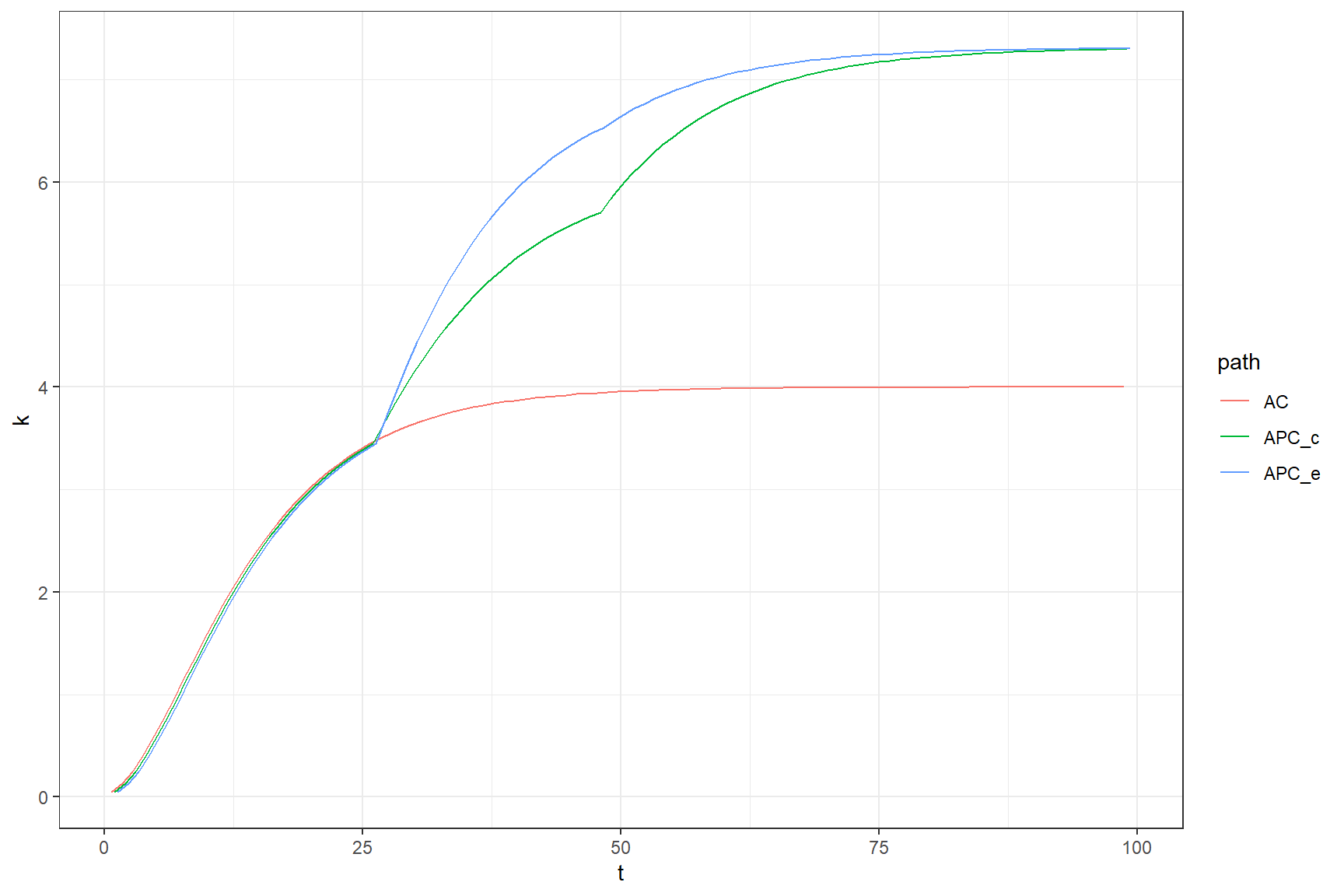Capital accumulation with violence as an option for the enforcer, $$|\delta, c$$ for $$v$$ = 0.2 $$c$$ = 0.4 $$d$$ = 0.2 $$s$$ = 0.4 , $$\alpha$$ = .5, $$A_{coop}$$ = 1.5, $$A_{nocoop}$$ = 1, $$A_{defect}$$ = 1.4

## 2.2$$\delta, c$$ graph

• Many paths, some with ultimate income convergence, some with permanent inequality
## build the dataset
graph_data <- data.frame(c=numeric(), delta=numeric(), t=numeric(), k=numeric(), u=numeric(), eq=character())

for(c in .c_range) {
for(delta in .delta_range){
graph_data <-
paths(delta = delta, c = c) %>%
bind_rows(graph_data,.)
}}

write_rds(graph_data, "graph_data_2.rds")
graph_data <- read_rds("graph_data_2.rds")
## graph the results
graph_data %>% filter(t < 100) %>% filter(path %in% c("AC",  "APC_c",  "APC_e")) %>%
ggplot(aes(t,  k, color=path)) + geom_line(position = position_dodge(0.9)) + facet_grid(c ~  delta , labeller = label_both) +
theme_bw()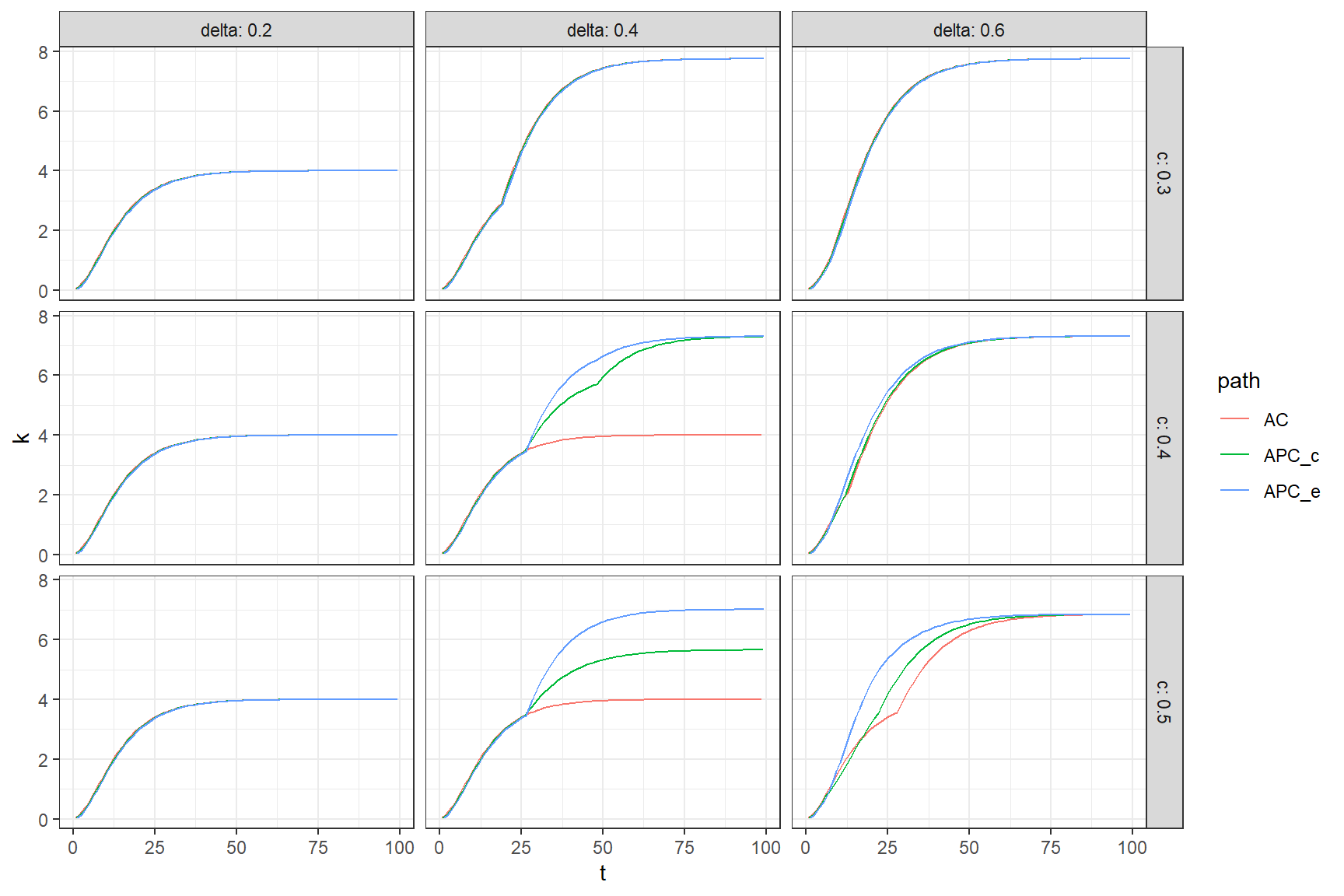Capital accumulation with violence as an option for the enforcer, $$|\delta, c$$ for $$v$$ = $$c-0.1$$, $$d$$ = 0.2 $$s$$ = 0.4 , $$\alpha$$ = .5, $$A_{coop}$$ = 1.5, $$A_{nocoop}$$ = 1, $$A_{defect}$$ = 1.4

pdf("main.pdf", width = 6, height = 4)
graph_data %>% filter(t < 100) %>% filter(path %in% c("AC",  "APC_c",  "APC_e")) %>% filter(delta == .4, c == .4) %>%
ggplot(aes(t,  k, color=path)) + geom_line() +
theme_bw() + xlab("Time") + ylab("Capital")
dev.off()
## png
##   2
pdf("main_bw.pdf", width = 6, height = 4)
graph_data %>% filter(t < 100) %>% filter(path %in% c("AC",  "APC_c",  "APC_e")) %>%
mutate(path = factor(path, c("AC",  "APC_c",  "APC_e"), c("AC",  "APC[c]",  "APC[e]"))) %>%
filter(delta == .4, c == .4) %>%
ggplot(aes(t,  k, linetype =path)) + geom_line() +
theme_bw() + xlab("Time") + ylab("Capital") +
scale_linetype_discrete(labels = scales::parse_format())
dev.off()
## png
##   2

## 2.3 Utility figure (with shocks)

Utility can take a drop or jump in transition periods as players start paying for public goods in anticipation of future gains. Bigger drops when delta is large. Bigger drops for transition to violence than to natural transition.

## graph the results
graph_data %>% filter(t < 100) %>% filter(path %in% c("AC",  "APC_c",  "APC_e")) %>%
ggplot(aes(t,  u, color=path)) + geom_line(position = position_dodge(0.9)) + facet_grid(c ~  delta , labeller = label_both) +
theme_bw()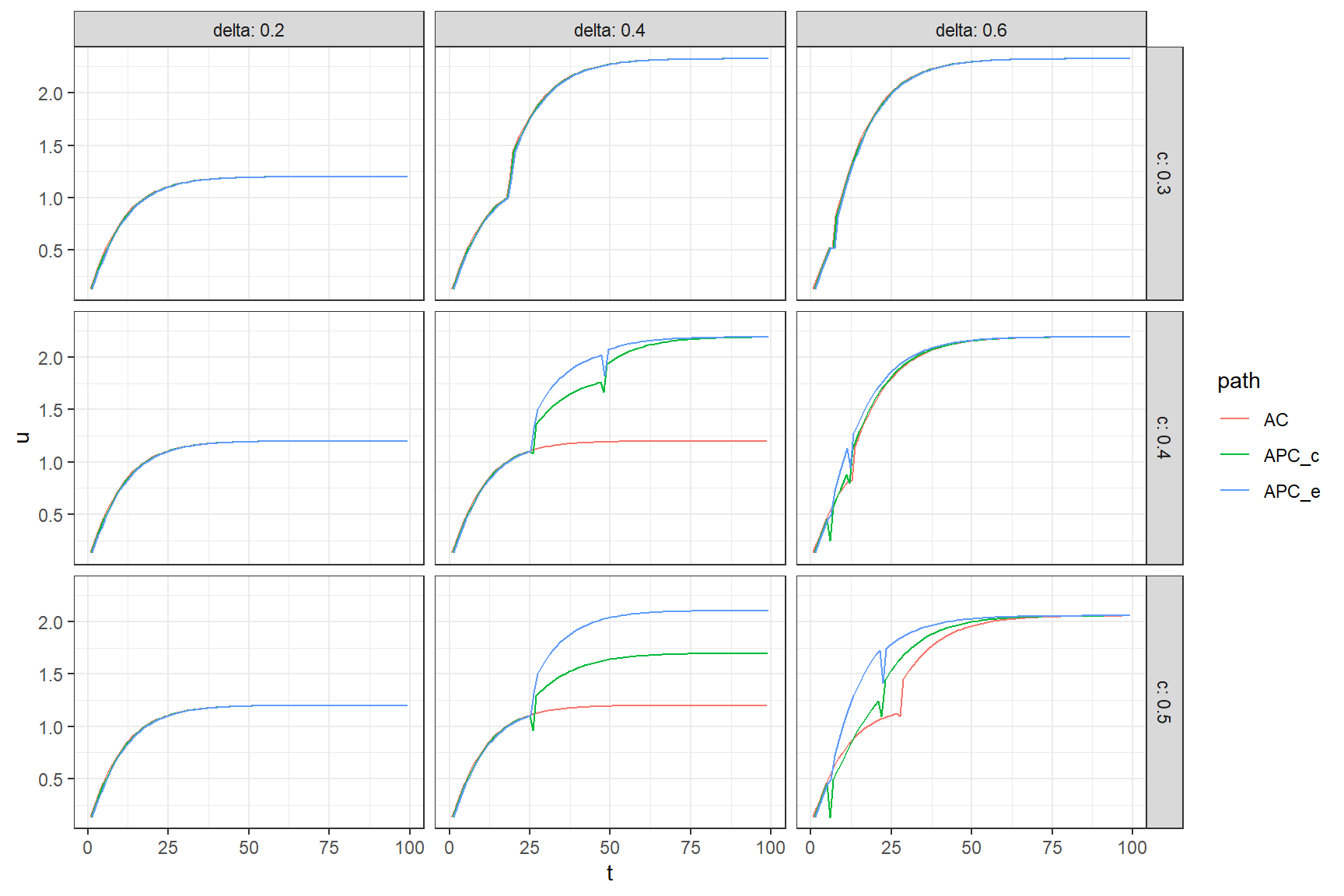Utility

## 2.4 Permanent violence or temporary

Citizens prefer a temporary violence equilibriunm when available Agents sometimes prefer a temporary violence equilibrium and sometimes permanent violence. This likely depends on the extent to which they would gain from their own returns to investing in public goods give their capital.

## graph the results
graph_data %>% filter(t < 100) %>% filter(path %in% c("AP_c", "AP_e", "APC_c",  "APC_e")) %>%
ggplot(aes(t,  k, color=path)) + geom_line(position = position_dodge(0.9)) + facet_grid(c ~  delta , labeller = label_both) +
theme_bw()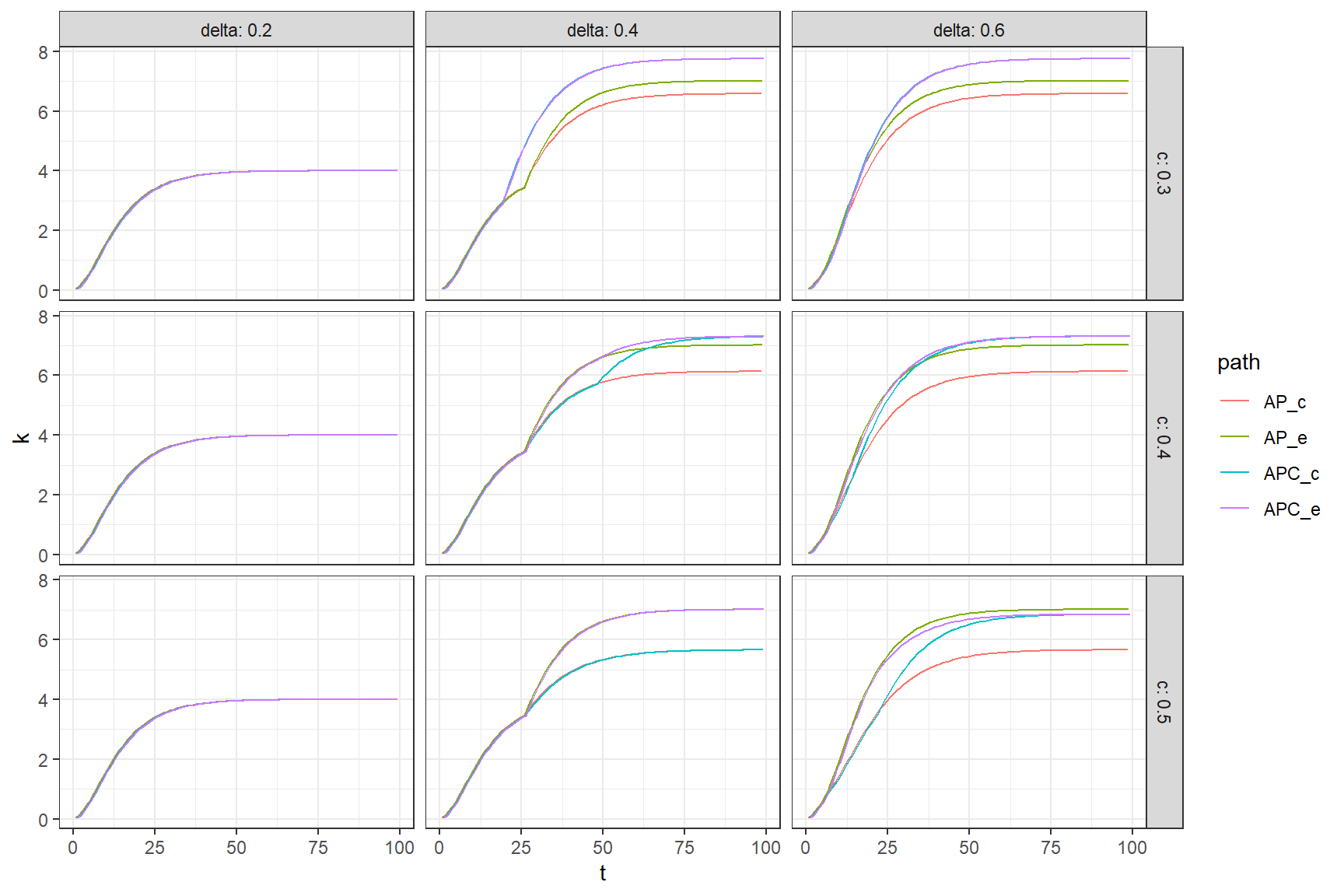Returns to temporary or permanent violence equilibria.

cutoffs <- find_cutoff_start(delta = .2, fine = .1, c = .4)
cutoffs\$transitions
##  cut_transition_to_temp_violence       cut_transition_to_all_coop
##                              Inf                              201
## cut_transition_to_all_coop_check  cut_transition_to_perm_violence
##                              Inf                              Inf
main_3 <- paths(delta = .2, c = .4)
write_rds(main_3, "single_graph_data_3.rds")

## graph the results
read_rds("single_graph_data_3.rds") %>% filter(t < 100) %>% #filter(path %in% c("AC",  "APC_c",  "APC_e")) %>%
ggplot(aes(t,  k, color=path)) + geom_line(position = position_dodge(0.9)) +   theme_bw()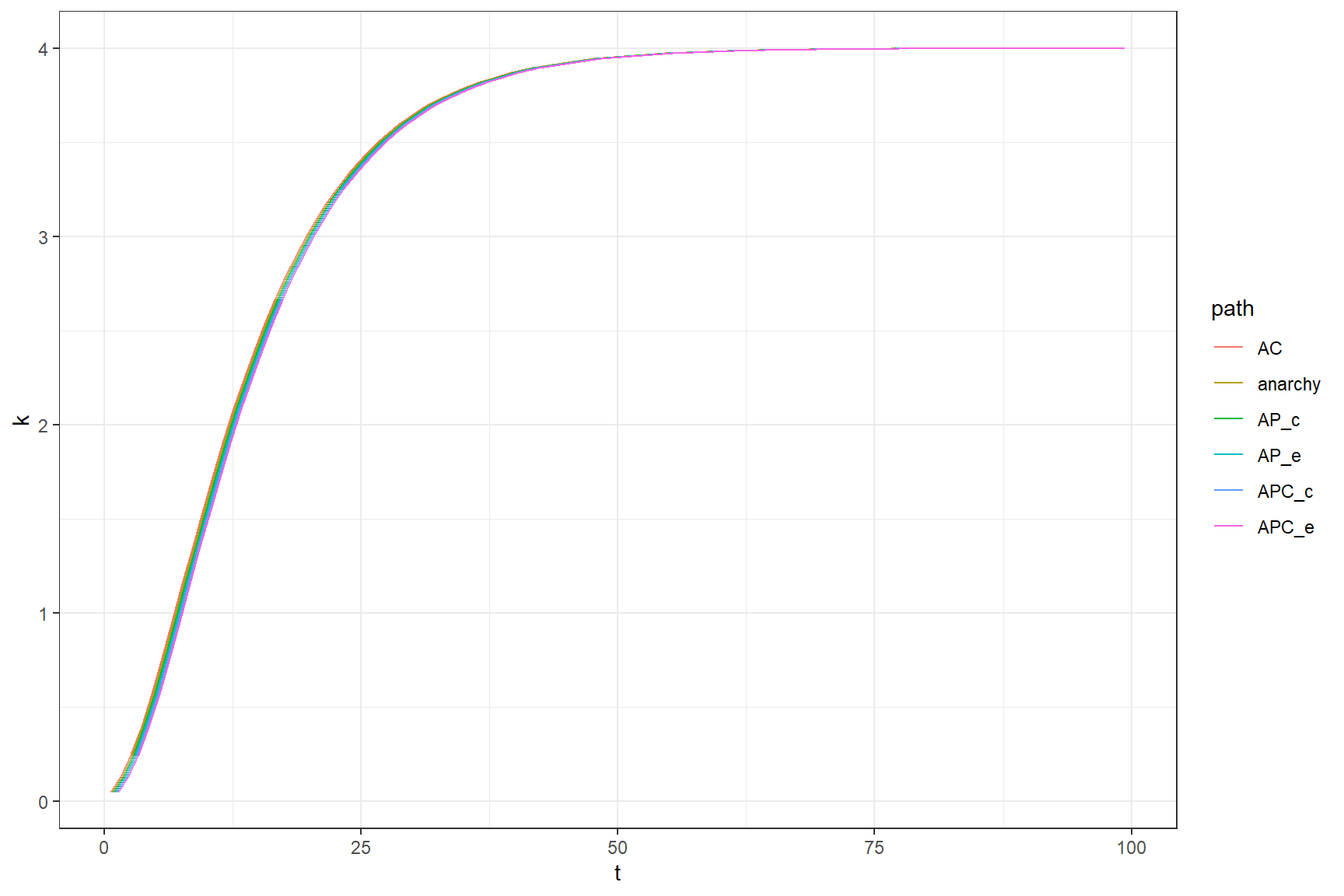Confusing case

## build the dataset

graph_data2 <- data.frame(c=numeric(), delta=numeric(), t=numeric(), k=numeric(), u=numeric(), eq=character())

for(c in .c_range) {
for(v in .v_range){
graph_data2 <-
paths(v = v, c = c) %>%
bind_rows(graph_data2,.)
}}

graph_data2 <- graph_data2 %>%
mutate(c = factor(c, labels = c(
expression(c[a]*" = 0.3"),
expression(c[a]*" = 0.4"),
expression(c[a]*" = 0.5")))) %>%
mutate(v = factor(v, labels = c(
expression(c[v]*" = 0.0"),
expression(c[v]*" = 0.2"),
expression(c[v]*" = 0.4"))))

write_rds(graph_data2, "graph_data2_2.rds")
graph_data2 <- read_rds("graph_data2_2.rds")

## 2.5 The costs of violence (Figure in text)

• Note when costs are low enough inequality can be so high that convergence is two sided
costs_graph <-
graph_data2 %>% filter(t < 100) %>% filter(path %in% c("AC",  "APC_c",  "APC_e")) %>%
mutate(path = factor(path, c("AC",  "APC_c",  "APC_e"), c("AC",  "APC[c]",  "APC[e]"))) %>%
ggplot(aes(t,  k, linetype=path)) + geom_line(position = position_dodge(0.9)) + facet_grid(c ~  v ,
labeller = label_parsed
) + xlab("Time") +  ylab("Capital") + theme_bw()  +
scale_linetype_discrete(labels = scales::parse_format())
## graph the results
costs_graph